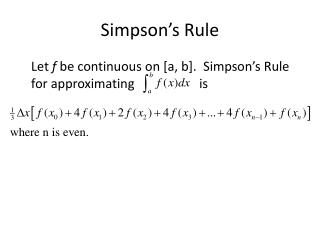DownloadDownload PresentationSimpson’s Rule

# Simpson’s Rule

Download Presentation## Simpson’s Rule

- - - - - - - - - - - - - - - - - - - - - - - - - - - E N D - - - - - - - - - - - - - - - - - - - - - - - - - - -
##### Presentation Transcript

1. Simpson’s Rule Let f be continuous on [a, b]. Simpson’s Rule for approximating is

2. Deriving Simpson’s Rule • Remember from Algebra 2 that you can create a parabola through any three given points. • If we choose two consecutive subintervals and the three corresponding points on the function, then we can create a parabola through those points.

3. We could then find the area under the parabola to approximate the area under the curve over the two subintervals.

4. Just like in Algebra 2, substitute each point into the general form of a quadratic equation to create a system of three equations.

5. Simplify by solving the system: Now integrate

6. Therefore, since [-h, h] is really [x0, x2] and h = x, then can be changed into

7. Applying Simpson’s Rule below from x0 to x6 we get Generalizing Simpson’s Rule, we get

8. Example Approximate using Simpson’s Rule if n = 4.